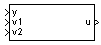Main Content

# 2D Controller Blend

Implement 2-D vector of state-space controllers by linear interpolation of their outputs

• Library:
• Aerospace Blockset / GNC / Control

•## Description

The 2D Controller Blend block implements an array of state-space controller designs. The controllers are run in parallel, and their outputs interpolated according to the current flight condition or operating point. The advantage of this implementation approach is that the state-space matrices A, B, C, and D for the individual controller designs do not need to vary smoothly from one design point to the next. The output from this block is the actuator demand, which you can input to an actuator block.

For the 2D Controller Blend block, at any given instant in time, nine controller designs are updated.

As the value of the scheduling parameter varies and the index of the controllers that need to be run changes, the states of the oncoming controller are initialized by using the self-conditioned form as defined for the Self-Conditioned [A,B,C,D] block.

## Limitations

This block requires the Control System Toolbox™ license.

## Ports

### Input

expand all

Aircraft measurements, specified as a vector.

Data Types: `double`

Scheduling variable, specified as a vector, that conforms to the dimensions of the state-space matrices.

Data Types: `double`

Scheduling variable, specified as a vector, that conforms to the dimensions of the state-space matrices.

Data Types: `double`

### Output

expand all

Actuator demands, specified as a vector.

Data Types: `double`

## Parameters

expand all

A-matrix of the state-space implementation. In the case of 2-D blending, the A-matrix should have four dimensions, the last two corresponding to scheduling variables v1 and v2. For example, if the A-matrix corresponding to the first entry of v1 and first entry of v2 is the identity matrix, then ```A(:,:,1,1) = [1 0;0 1];```.

#### Programmatic Use

 Block Parameter: `A` Type: character vector Values: vector Default: `'A'`

B-matrix of the state-space implementation. The B-matrix should have three dimensions, the last one corresponding to the scheduling variable v. For example, if the B-matrix corresponding to the first entry of v is the identity matrix, then ```B(:,:,1) = [1 0;0 1];```.

#### Programmatic Use

 Block Parameter: `B` Type: character vector Values: vector Default: `'B'`

C-matrix of the state-space implementation. The C-matrix should have three dimensions, the last one corresponding to the scheduling variable v. For example, if the C-matrix corresponding to the first entry of v is the identity matrix, then ```C(:,:,1) = [1 0;0 1];```.

#### Programmatic Use

 Block Parameter: `C` Type: character vector Values: vector Default: `'C'`

D-matrix of the state-space implementation. The D-matrix should have three dimensions, the last one corresponding to the scheduling variable v. For example, if the D-matrix corresponding to the first entry of v is the identity matrix, then ```D(:,:,1) = [1 0;0 1];```.

#### Programmatic Use

 Block Parameter: `D` Type: character vector Values: vector Default: `'D'`

Breakpoints for the first scheduling variable, specified as a vector. The length of v1 should be same as the size of the third dimension of A, B, C, and D.

#### Programmatic Use

 Block Parameter: `breakpoints_v1` Type: character vector Values: vector Default: `'v1_vec'`

Breakpoints for the second scheduling variable, specified as a vector. The length of v2 should be same as the size of the fourth dimension of A, B, C, and D.

#### Programmatic Use

 Block Parameter: `breakpoints_v2` Type: character vector Values: vector Default: `'v2_vec'`

Vector of initial states for the controller, that is, initial values for the state vector, x. It should have length equal to the size of the first dimension of A.

#### Programmatic Use

 Block Parameter: `x_initial` Type: character vector Values: vector Default: `'0'`

For oncoming controllers, an observer-like structure is used to ensure that the controller output tracks the current block output, u. The poles of the observer are defined in this dialog box as a vector, the number of poles being equal to the dimension of the A-matrix. Poles that are too fast result in sensor noise propagation, and poles that are too slow result in the failure of the controller output to track u.

#### Programmatic Use

 Block Parameter: `vec_w` Type: character vector Values: vector Default: ```'[-5 -2]'```

 Hyde, R. A. “H-infinity Aerospace Control Design - A VSTOL Flight Application.” Springer Verlag: Advances in Industrial Control Series, 1995.

## SupportGet trial now